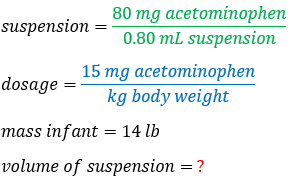# Problem: An acetaminophen suspension for infants contains 80 mg/0.80 mL suspension. The recommended dose is 15 mg/ kg body weight. How many mL of this suspension should be given to an infant weighing 14 lb? (Assume two significant figures.)

###### FREE Expert Solution
90% (374 ratings)
###### FREE Expert Solution

The volume of the suspension can be calculated using the concentration of the suspension given. However, we will have to convert some units in order to do the calculation

Given:When transforming one unit to another, recall the conversion factors and/or constants that will be used in the calculation. We will use the following conversion factors:

90% (374 ratings)###### Problem Details

An acetaminophen suspension for infants contains 80 mg/0.80 mL suspension. The recommended dose is 15 mg/ kg body weight. How many mL of this suspension should be given to an infant weighing 14 lb? (Assume two significant figures.)

What scientific concept do you need to know in order to solve this problem?

Our tutors have indicated that to solve this problem you will need to apply the Dimensional Analysis concept. You can view video lessons to learn Dimensional Analysis. Or if you need more Dimensional Analysis practice, you can also practice Dimensional Analysis practice problems.

What is the difficulty of this problem?

Our tutors rated the difficulty ofAn acetaminophen suspension for infants contains 80 mg/0.80 ...as low difficulty.

How long does this problem take to solve?

Our expert Chemistry tutor, Jules took 3 minutes and 30 seconds to solve this problem. You can follow their steps in the video explanation above.

What professor is this problem relevant for?

Based on our data, we think this problem is relevant for Professor Michael's class at UNC.

What textbook is this problem found in?

Our data indicates that this problem or a close variation was asked in Chemistry: A Molecular Approach - Tro 2nd Edition. You can also practice Chemistry: A Molecular Approach - Tro 2nd Edition practice problems.